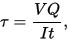# Shear stress in beam

## Homework Statementwhere

V = total shear force at the location in question;
Q = statical moment of area;
t = thickness in the material perpendicular to the shear;
I = Moment of Inertia of the entire cross sectional area.
based on this formula , the shear stress is max at the neutral axis of beam .... Why it is so ? Can someone prove me ?